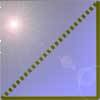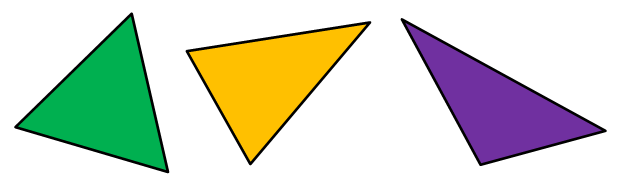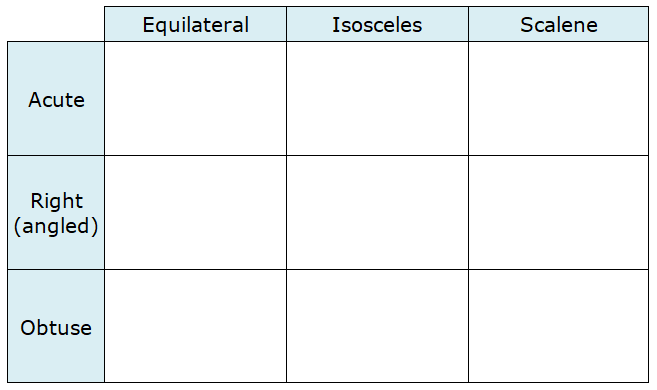#### You may also like### Tri.'s

How many triangles can you make on the 3 by 3 pegboard?### Cutting Corners

Can you make the most extraordinary, the most amazing, the most unusual patterns/designs from these triangles which are made in a special way?### Bracelets

Investigate the different shaped bracelets you could make from 18 different spherical beads. How do they compare if you use 24 beads?

# Name That Triangle!

## Name That Triangle!

What different types of triangle can you think of?See how many you can name, then click on the buttons below to find out what some other children have said.

Edd said:

"There are four types of triangle: equilateral, isosceles, scalene and right-angled."

Fleur said:

"There are only three types of triangle. These are equilateral, isosceles and scalene. Right-angled triangles don't count because all right-angled triangles are also isosceles or scalene triangles."

Harmony said:

"You can name a triangle using either the sides or the angles of the triangle. Using the sides, you can have equilateral, isosceles and scalene triangles. Using the angles, you get right-angled, acute or obtuse triangles."

Which of these children do you agree with? Why?

We normally name triangles by thinking about the lengths of the sides, but in this activity we will also be naming triangles using their angles. How could we do this? What do you think an 'acute triangle' or an 'obtuse triangle' might look like?

Have a look at the grid below.This grid is for matching up the different names for a triangle. For example, the box at the top on the left is for equilateral acute triangles - these are acute triangles with all three sides the same length.

Look at each space on the grid and try to sketch some different triangles to go in the box.
Can you sketch a triangle for every box in the grid? Why/why not?

### Why do this problem?

This activity provides an engaging context for children to deepen their understanding of the ways of naming different types of triangles. Children are often taught about four types of triangles (equilateral, isosceles, scalene and right-angled) in isolation from each other and they may be curious to learn that this is not the whole story. We should be aiming for learners to be comfortable with the different names a triangle can have and to explore when these definitions can overlap. Discussing the characteristics of the triangles with others will improve pupils' shape vocabulary as there becomes a need to communicate efficiently.

### Possible approach

Begin by discussing what pupils already know about naming triangles. How many types of triangle do children think there are? Can any triangle be more than one of these types? (Children might notice that right-angled triangles can be either isosceles or scalene.) Go through the different ideas under the 'show' buttons and give pupils the opportunity to support or challenge these ideas, giving reasons for what they think.

Recap on different types of angles and ask children what they think 'acute' and 'obtuse' triangles are. At this point in the lesson, pupils could be asked to draw some different examples of acuse, obtuse and right triangles and to explore whether or not a triangle can fall into more than one of these categories. Discuss the choice of names - why does an acute triangle need to have three acute angles, but an obtuse triangle only needs one obtuse angle? (Pupils should be encouraged to reason that at least two angles of a triangle always have to be acute, so we are only interested in what the third type of angle is. Challenging pupils to draw a picture of a triangle with two right angles or obtuse angles will help them grasp this concept.)

Once the different ways of naming a triangle have been discussed, give children the table (wordpdf) and ask them to work in pairs to draw at least one triangle in each box. If they believe it is impossible to draw a triangle for a particular box, ask them to write a sentence explaining why they think this is the case.

Bring the class back together when all pairs have finished, and discuss different triangles that pupils drew in each box. Which boxes can't have any triangles in them? Encourage children to articulate why they think this, building on each other's explanations.

### Key questions

How many sides of the same length do you need?
How big will each angle be?
Can you find a triangle that goes in that box? Why not?

### Possible extension

Children can see how many different triangles they can draw in each box - for example, all of the isosceles right-angled triangles will be similar triangles.

### Possible support

Some children might find it simpler to cut out the triangles from this sheet (wordpdf) and put these into the table rather than drawing their own.  It might be appropriate to have a go at the task Triangle or No Triangle? before tackling this one.